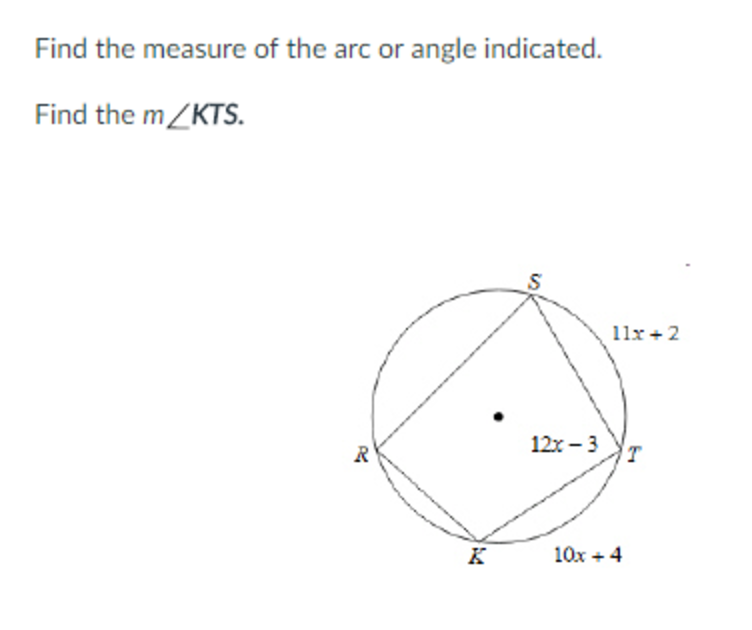D

#### How to solve for inscribed angle?

26 viewed last edited 6 years agoHanna Owens
2http://prntscr.com/ftomdf I'm not sure how to approach this problem. Would I first do 180 - (12x-3)? What do I do with the two arc lengths that are given?Vivekanand Vellanki
0You know the length of the arc KTS = 21x + 6. If the angle subtended by an arc at the center of the circle is y radians, the length of the arc = y*r. Assuming a unit circle, the angle subtended by KTS at the center is 21x + 6 radians. The angle KRS = 1/2(21x + 6). In a cyclic quadrilateral, the opposite angles add up to 180 degree, or pi radians. So, 12x-3 + (21x+6)/2 = \pi 22.5x=\pi